CipherApr 7, 2017

CNN - Sweden - truck rams into pedestrians - False Flag attack - Trump connections

http://edition.cnn.com/2017/04/07/europe/stockholm-truck-crash/index.html
Today is 4/7/2017 and apparently there was a "terrorist attack" in Sweden that is very much connected to Trump, the Prime Minister of Sweden and the King of Sweden. Before I start decoding this story from CNN I have to mention that Trump made up a terrorist attack on Sweden at one of his rallies on 18th of February
2/18/2017 = 2+1+8+2+0+1+7 = 21
4/7/2017 = 4+7+2+0+1+7 = 21
Sweden = 1+5+5+4+5+5 = 25 (Full Reduction)
Trump = 2+9+3+4+7 = 25 (Full Reduction)
This is happening on 97th day of the year (25th prime number is 97)

On 2/19/2017 mainstream media started covering Trump's false claims, measuring from 2/19 to 4/7, we find 47 days
Stockholm, Sweden = 8+7+12+24+16+19+12+15+14 + 8+4+22+23+22+13 = 219 (Reverse Ordinal)
---Update - 4/8/2017 ---
Prosecutor Hans Ihrman said the arrested man was a 39-year-old from Uzbekistan. The attack Friday in the heart of Sweden's capital killed four people and injured about 15 more.
four dead = 6+60+300+90 + 4+5+1+4 = 470 (English Extended
four dead = 6+15+21+18 + 4+5+1+4 = 74 (English Ordinal)
fifteen injured = 6+9+6+2+5+5+5 + 9+5+1+3+9+5+4 = 74 (Full Reduction)
fifteen injured = 6+9+6+100+5+5+40 + 9+40+600+200+80+5+4 = 1109 (Jewish)
Now they are saying that suspect is from Uzbekistan = 3+8+2+5+2+9+10+2+1+5 = 47 (Single Reduction)

Trump = 7+9+6+14+11 = 47 (Reverse Ordinal)
President = 7+9+5+1+9+4+5+5+2 = 47 (Full Reduction)
Now they are saying that suspect is from Uzbekistan = 3+8+2+5+2+9+10+2+1+5 = 47 (Single Reduction)

A man arrested on suspicion of terrorism offenses after the Stockholm truck attack is from the central Asian republic of Uzbekistan and was known to intelligence services, Swedish authorities said.

Also note that this is coming 1 month and 19 days after Trump made up the attack (Trump was announced as the winner of 2016 elections on 11/9/2016 - 314th day of the year) - Donald = 4+50+40+1+20+4 = 119 (Jewish)Mr. Trump = 13+18 + 20+18+21+13+16 = 119 (English Ordinal)

There seems to be Pi coding following Trump and this time 314 connects to the Prime Minister of Sweden Stefan LofvenNote that he's the 33rd Prime Minister of Sweden and his full name sums to 90 (one of two numbers that sum to 33 when written out)
Kjell Stefan Lofven = 7+8+4+6+6 + 8+7+4+3+8+4 + 6+3+3+5+4+4 = 90 (Reverse Reduction)
Ninety = 5+9+5+5+2+7 = 33 (Full Reduction)
"False flag" = 6+1+3+1+5 + 6+3+1+7 = 33 (Full Reduction)

From 4/7/2017 to the birthday of Prime Minister of Sweden is 105 days (3 months and 14 days) - 105 is also interesting considering the fact that mainstream media started covering Trump's false claims on "Nineteenth of February" = 5+9+5+5+2+5+5+5+2+8 + 6+6 + 6+5+2+9+3+1+9+7 = 105 (Full Reduction)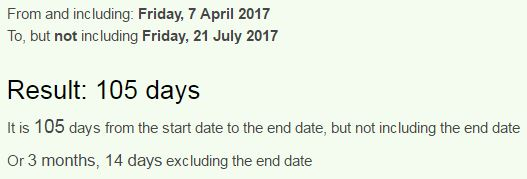They are saying that "Hijacked truck" rammed into pedestrians
hijacked truck = 19+18+17+26+24+16+22+23 + 7+9+6+24+16 = 227 (Reverse Ordinal)
22/7 = 3.14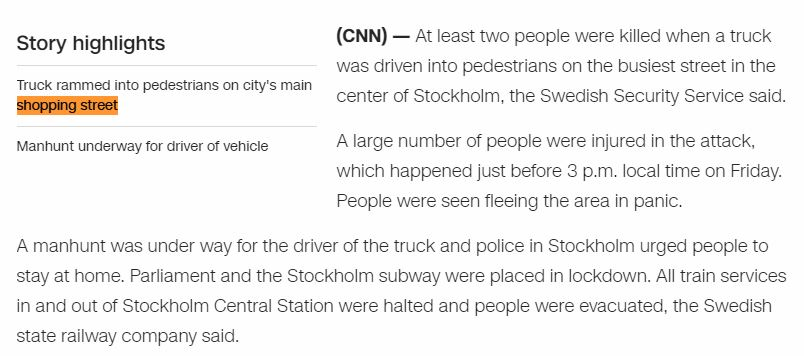This attack happened in main "shopping street" = 1+8+6+7+7+9+5+7 + 1+2+9+5+5+2 = 74 (Full Reduction)
This date is written 7/4 in Sweden
"Killing" = 11+9+12+12+9+14+7 = 74 (English Ordinal)

"Sweden" = 90+900+5+4+5+40 = 1044 (Jewish)
"Stockholm" = 10+2+6+3+2+8+6+3+4 = 44 (Single Reduction)
"Forty four" = 6+15+18+20+25 + 6+15+21+18 = 144 (English Ordinal)
Here's the connection to the King of Sweden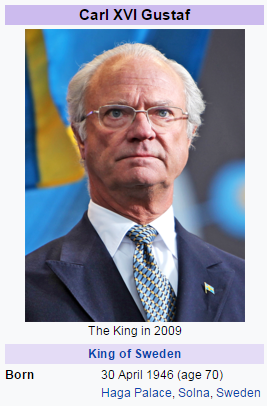30th of April is birthday of the King of Sweden and it's interesting that his birthday is coming on a date with 44 numerology this year
4/30/2017 = 4+30+2+0+1+7 = 44
He's celebrating his 71st birthday, 44 and 71 are the only two numbers that sum to 144 when written out
Seventy one = 19+5+22+5+14+20+25 + 15+14+5 = 144 (English Ordinal)

From his last birthday to this attack is 343 days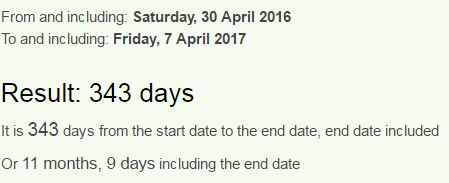Also note 11 months and 9 days and recall how this is coming 1 month and 19 days after Trump made up a terrorist attack on Sweden...343 = 7*7*7
22/7 = 3.14
Twenty two divided by seven = 20+23+5+14+20+25 + 20+23+15 + 4+9+22+9+4+5+4 + 2+25 + 19+5+22+5+14 = 314 (English Ordinal)
Seven hundred seventy seven = 19+5+22+5+14 + 8+21+14+4+18+5+4 + 19+5+22+5+14+20+25 + 19+5+22+5+14 = 314 (English Ordinal)

King of Sweden is celebrating his 71st birthday on the 24th day from the attack
Twenty four = 200+500+5+50+200+700 + 6+60+300+90 = 2111 (English Extended)7/22 = 0.318Note 3 weeks and 3 days, a lot like 33, and it's very interesting how we have 33rd prime minister of Sweden and Carl the 16th connecting to this story
Sixteen = 1+9+6+2+5+5+5 = 33 (Full Reduction)
But there are even more 33's and it's getting really funnyThey actually didn't spell his name right, and it might even be on purpose.. if you go to Wikipedia it's showing Gustav Fridolin, if we spell his name like the CNN did, it comes up to 71 connecting back to 44
Gustaf Fridolin = 7+3+1+2+1+6 + 6+9+9+4+6+3+9+5 = 71 (Full Reduction)
Gustaf = 7+200+90+100+1+6 = 404 (Jewish)
I guess it's very easy to see why they misspelled his name...
Also he's born on Tenth of May = 7+4+4+7+1 + 3+3 + 5+8+2 = 44 (Reverse Reduction)When we measure from the attack to his next birthday we find something even more interesting
First note that he's currently 33 years old and this attack took place 33 days before his birthday... Nothing suspicious here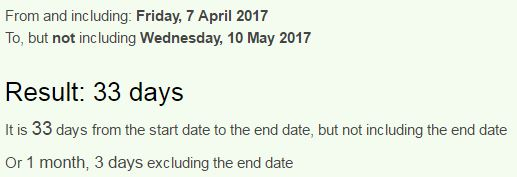4/9/2017 - CNN updated the story once againThis will change things = 20+8+9+19 + 23+9+12+12 + 3+8+1+14+7+5 + 20+8+9+14+7+19 = 227 (English Ordinal